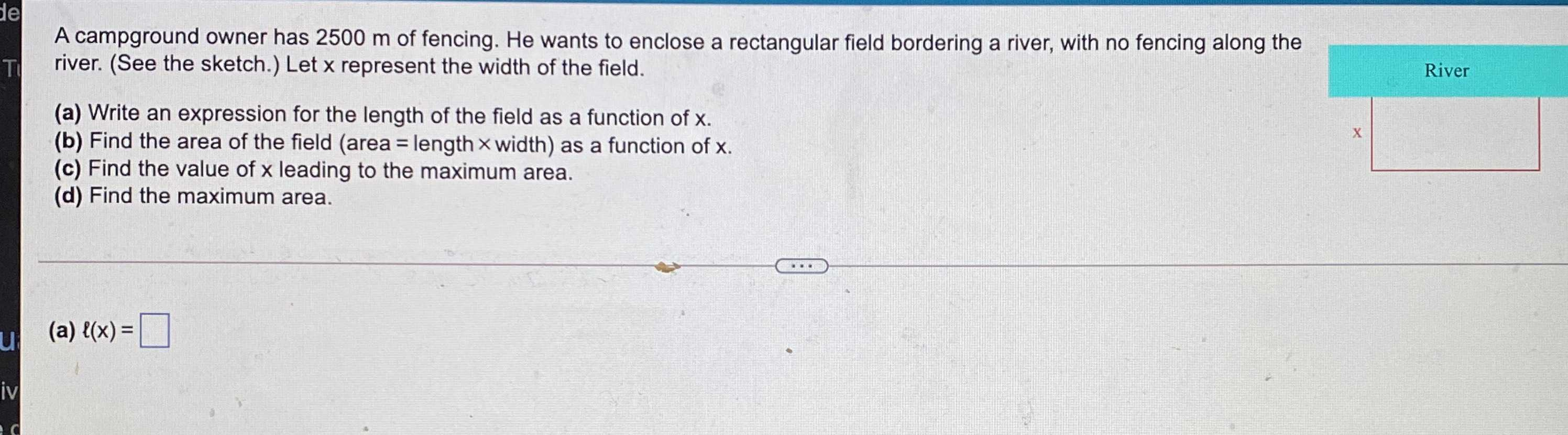### ¿Todavía tienes preguntas de matemáticas?

Pregunte a nuestros tutores expertos
Algebra
PreguntaA campground owner has $$2500$$ m of fencing. He wants to enclose a rectangular field bordering a river, with no fencing along the river. (See the sketch.) Let $$x$$ represent the width of the field.

(a) Write an expression for the length of the field as a function of $$x$$ . (b) Find the area of the field (area = length $$x$$ width) as a function of $$x$$ .

(c) Find the value of $$x$$ leading to the maximum area.

(d) Find the maximum area. (a) $$\ell ( x ) = \square$$